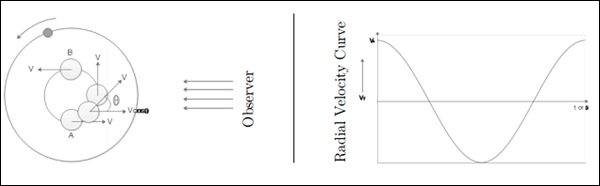# Cosmology - Extrasolar Planet Detection

Astrobiology is the study of origin, evolution, distribution and future of life in the universe. It is concerned with discovering and detecting Extrasolar Planets.

Astrobiology addresses the following points −

• How does life begin and evolve? (biology + geology + chemistry + atmospheric sciences)

• Are there worlds beyond earth that are favorable for life? (astronomy)

• What would be the future of life on earth?

Astronomy addresses the following points −

• How to detect the planetary system around other stars?

• One of the method is direct imaging, but it is a very difficult task because planets are extremely faint light sources compared to stars, and what little light comes from them tends to be lost in the glare from their parent star.

• Contrast is better when the planet is closer to its parent star and hot, so that it emits intense infrared radiation. We can make images in the infrared region.

## Techniques for Extrasolar Planet Detection

The most efficient techniques for extrasolar planet detection are as follows. Each of these are also explained in detail in the subsequent chapters.

It is also called as the Doppler method. In this −

• The star planet system revolves around their barycenter, star wobbles.

• Wobbling can be detected by

• Periodic Red/Blue shifts. Astrometry - measuring the objects in the sky very precisely.

### Transit Method

Transit Method (Kepler space telescope) is used to find out the size. The dip in brightness of star by planet is usually very less, unlike in a binary system.

### Direct Imaging

Imaging the planet using a telescope.

Let us look at a case study done on Radial Velocity Method.

## Case Study

This case study is on the Circular orbit and the plane of the orbit perpendicular to the plane of the sky. The time taken by both around the barycenter will be same. It will be equal to the time difference between two Redshift or Blueshift.

Consider the following image.At A and C – full velocity is measured. At C, velocity is zero.

• Vrmax = V* is the true velocity of the star.

• P is the time-period of the star as well as the planet.

• θ is the phase of orbit.

• Star Mass - M*, Orbit radius a*, planet mass mp.

From center of mass equation,

$$m_p a_p = M_\ast a_\ast$$

From equation of velocity,

$$V_\ast = \frac{2\pi a_\ast}{P}$$

$$\Rightarrow a_\ast = \frac{PV_\ast}{2\pi}$$

From Kepler’s Law,

$$P^2 = \frac{4\pi^2a_p^3}{GM_\ast}$$

$$\Rightarrow a_p = \left ( \frac{P^2GM_\ast}{4\pi^2} \right)^{1/3}$$

From the above equations, we get −

$$\Rightarrow m_p = \left( \frac{P}{2\pi G} \right)^{1/3}M_\ast^{2/3}V_\ast$$

We get: $m_p, a_p$ and $a_\ast$.

The above equation is biased towards most massive planets close to the star.

### Points to Remember

• Astrobiology is the study of origin, evolution, distribution and future of life in the universe.

• Techniques to detect the extrasolar planets are: Radial Velocity Method, Transit Method, Direct Imaging, etc.

• Wobbling can be detected by periodic red/blue shifts and Astrometry.

• The Radial Velocity Method is biased towards detecting massive planets close to the star.